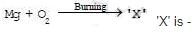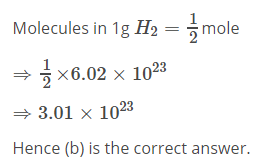Courses

# Test: Atoms And Molecules - 4

## 20 Questions MCQ Test NCERT Textbooks (Class 6 to Class 12) | Test: Atoms And Molecules - 4

Description
This mock test of Test: Atoms And Molecules - 4 for UPSC helps you for every UPSC entrance exam. This contains 20 Multiple Choice Questions for UPSC Test: Atoms And Molecules - 4 (mcq) to study with solutions a complete question bank. The solved questions answers in this Test: Atoms And Molecules - 4 quiz give you a good mix of easy questions and tough questions. UPSC students definitely take this Test: Atoms And Molecules - 4 exercise for a better result in the exam. You can find other Test: Atoms And Molecules - 4 extra questions, long questions & short questions for UPSC on EduRev as well by searching above.
QUESTION: 1

### The elements present in baking soda are -

Solution: Baking soda, also called sodium bicarbonate, is a common baking ingredient, cleaner, deodorizer and pH regulator. It is commonly sold as a white powder that looks similar to baking powder. Unlike baking powder, which contains acidic ingredients, however, baking soda is a single compound composed of only four elements: sodium, hydrogen, carbon and oxygen.
QUESTION: 2

### The first scientist to use of the symbols of elements was-

Solution:
John Dalton
John Dalton was the first scientist to use symbols for elements. He was first to use chemical symbols for elements perfectly in his table of elements , which made chemical chemistry easy.
QUESTION: 3

### The overall charge on an ionic compound is equal to -

Solution:
QUESTION: 4

The chemical formula of the copper nitrate -

Solution: Copper nitrate, also known as Cupric nitrate and its chemical formula is Cu(NO3)2
QUESTION: 5

The number of carbon atoms in 1g of CaCO3 is-

Solution:
QUESTION: 6

The mass of a single atom of carbon is -

Solution:
QUESTION: 7

The mass of 1 u is -

Solution:
QUESTION: 8

How many molecules are present in 9g of water -

Solution:
No. of molecules in 9g of water:

Molecular mass of water(H2O)= (1x2)+16=18g/mol

1 mole of water=18g of water contains=6.022x10^23 molecules of water

then 9g of water will have= (6.022x10^23/18)x9 = 3.011x10^23 molecules
QUESTION: 9Solution:
QUESTION: 10

The formula of sulphuric acid is-

Solution:
QUESTION: 11

Which of the following has largest number of particles?

Solution:
QUESTION: 12

Mass of one Avogadro's number of O atom is equal to -

Solution: 1 mole of any atom is = 6.022 x 10 ^23 atom ( avogadro's number ) now, there are Avogadro's number of O atom, which will be = 1 mole ie ; we have 1 mole oxygen atom . mole = weight / molecular weight 1 = weight / 16 ( molecular weight of O = 16 a.m.u ) weight = 16 g ( answer )
QUESTION: 13

How many molecules are present in one gram of hydrogen gas?

Solution:QUESTION: 14

The correct formula of aluminium sulphate is

Solution:
QUESTION: 15

Which of the following has highest intermolecular forces of attraction -

Solution: As the carbon dioxide is in solid form(dry ice) so it has gained all the properties of solid i.e. now they have highest intermolecular force between them.
QUESTION: 16

Which of the following is not correct regarding gases?

Solution:
QUESTION: 17

The Boiling point of water at normal atmospheric pressure is -

Solution:
QUESTION: 18

Avogadro's number represents the number, of atoms in-

Solution:
QUESTION: 19

Molecular mass of ozone is

Solution:
QUESTION: 20

Which of the following is not correct according to Dalton's atomic theory?

Solution: